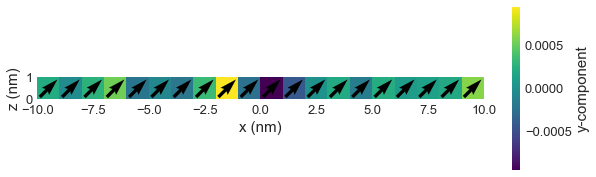# Adding multiple terms of the same class¶

Here we demonstrate how to have multiple energy terms of the same class in the energy equation. For the sample, we choose a one-dimensional chain of magnetic moments.

In :
import random
import oommfc as mc
import discretisedfield as df
import micromagneticmodel as mm

p1 = (-10e-9, 0, 0)
p2 = (10e-9, 1e-9, 1e-9)
cell = (1e-9, 1e-9, 1e-9)
region = df.Region(p1=p1, p2=p2)
mesh = df.Mesh(region=region, cell=cell)


The mesh is

In :
mesh.k3d()


The system has an energy equation, which consists of two Zeeman energy terms.

In :
H1 = (0, 0, 1e6)
H2 = (1e6, 0, 0)

system = mm.System(name='multiple_terms')


Now, if we try to add two energy terms, we get an exception raised.

In :
try:
system.energy = mm.Zeeman(H=(0, 0, 1e5)) + mm.Zeeman(H=(0, 1e5, 0))
except ValueError:
print('Exception raised.')

Exception raised.


This is because different energy terms must have different names, so they can be uniquely identified. So, we have to give names to our energy terms:

In :
system.energy = mm.Zeeman(H=H1, name='zeeman1') + mm.Zeeman(H=H2, name='zeeman2')


We are going to minimise the system's energy using oommfc.MinDriver later. Therefore, we do not have to define the system's dynamics equation. Finally, we need to define the system's magnetisation (system.m). We are going to make it random with $M_\text{s}=8\times10^{5} \,\text{Am}^{-1}$

In :
import random

random.seed(1)

Ms = 8e5  # saturation magnetisation (A/m)

def m_fun(pos):
return [2*random.random()-1 for i in range(3)]

system.m = df.Field(mesh, dim=3, value=m_fun, norm=Ms)


Now, we can minimise the system's energy by using oommfc.MinDriver.

In :
md = mc.MinDriver()
md.drive(system)

Running OOMMF (ExeOOMMFRunner) [2020/07/01 21:25]... (2.5 s)


We expect that now all magnetic moments are aligned parallel or antiparallel to the anisotropy axis (in the $z$-direction).

In :
system.m.plane('y').mpl(figsize=(10, 3))We can see that magnetisation is aligned with the sum of fields H1+H2. Finally, let us have a look at the table:

In :
system.table.data

Out:
max_mxHxm E delta_E bracket_count line_min_count conjugate_cycle_count cycle_count cycle_sub_count energy_calc_count E_zeeman1 E_zeeman2 iteration stage_iteration stage mx my mz
0 0.003334 -2.843445e-20 -7.222237e-35 27.0 5.0 21.0 23.0 2.0 33.0 -1.421723e-20 -1.421723e-20 30.0 30.0 0.0 0.707107 9.591067e-11 0.707107

We can see that energy terms are marked with the names we gave to energy terms when we defined the energy equation.